# Bayesian Theory and Bayesian networks 1 Probability All

• Slides: 24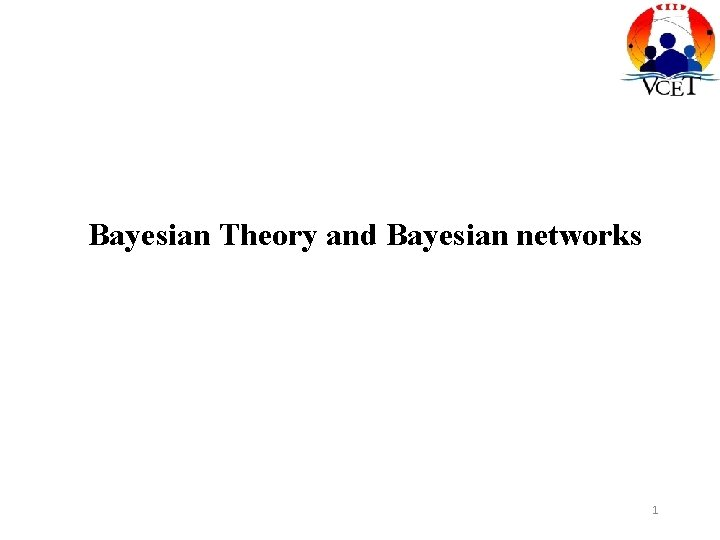Bayesian Theory and Bayesian networks 1Probability • All probabilities are between 0 and 1 • Necessarily true propositions have probability=1 and necessarily false propositions have probability=0 2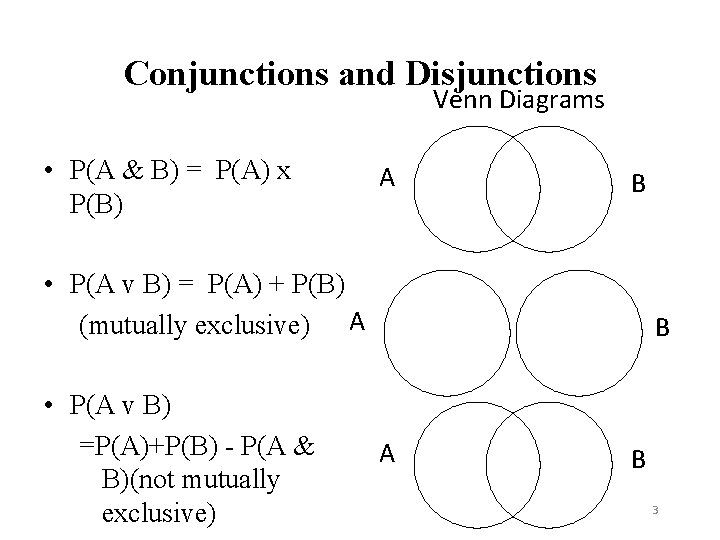Conjunctions and Disjunctions Venn Diagrams • P(A & B) = P(A) x P(B) A B • P(A v B) = P(A) + P(B) (mutually exclusive) A • P(A v B) =P(A)+P(B) - P(A & B)(not mutually exclusive) B A B 3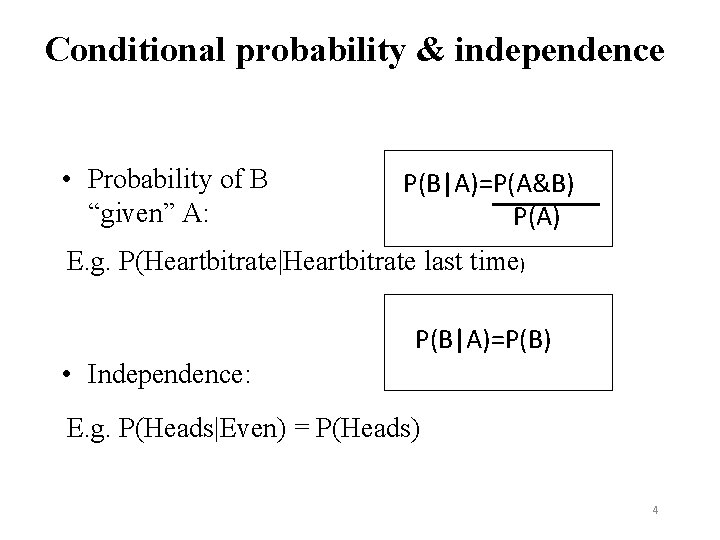Conditional probability & independence • Probability of B “given” A: P(B|A)=P(A&B) P(A) E. g. P(Heartbitrate|Heartbitrate last time) P(B|A)=P(B) • Independence: E. g. P(Heads|Even) = P(Heads) 4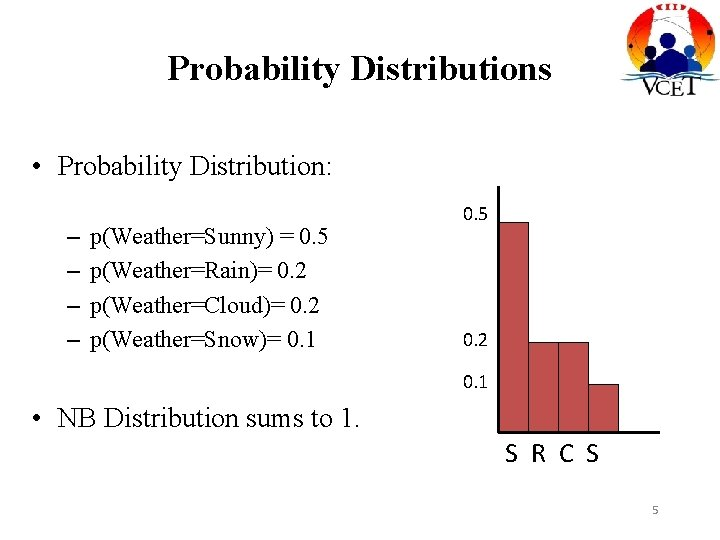Probability Distributions • Probability Distribution: – – p(Weather=Sunny) = 0. 5 p(Weather=Rain)= 0. 2 p(Weather=Cloud)= 0. 2 p(Weather=Snow)= 0. 1 0. 5 0. 2 0. 1 • NB Distribution sums to 1. S R C S 5Joint Probability • Completely specifies all beliefs in a problem domain. • Joint probability Distribution is an n-dimensional table with a probability in each cell of that state occurring. • Written as P(X 1, X 2, X 3 …, Xn) • When instantiated as P(x 1, x 2 …, xn) 6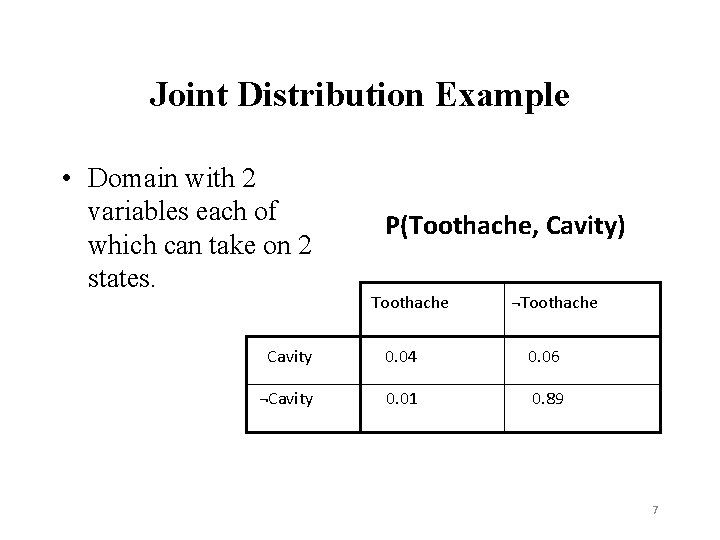Joint Distribution Example • Domain with 2 variables each of which can take on 2 states. P(Toothache, Cavity) Toothache ¬Toothache Cavity 0. 04 0. 06 ¬Cavity 0. 01 0. 89 7Bayes’ Theorem Simple: P(Y|X) = P(X|Y)P(Y) P(X) General: P(Y|X, E) = P(X|Y, E)P(Y|E) P(X|E) 8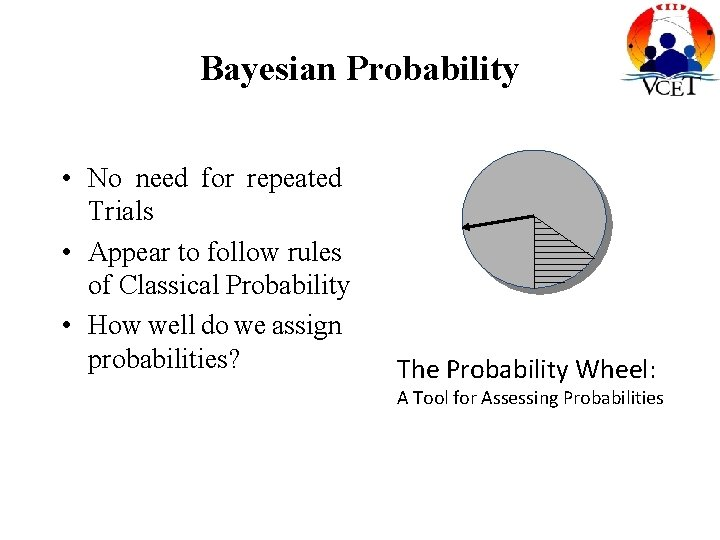Bayesian Probability • No need for repeated Trials • Appear to follow rules of Classical Probability • How well do we assign probabilities? The Probability Wheel: A Tool for Assessing ProbabilitiesOutline • Syntax • Semantics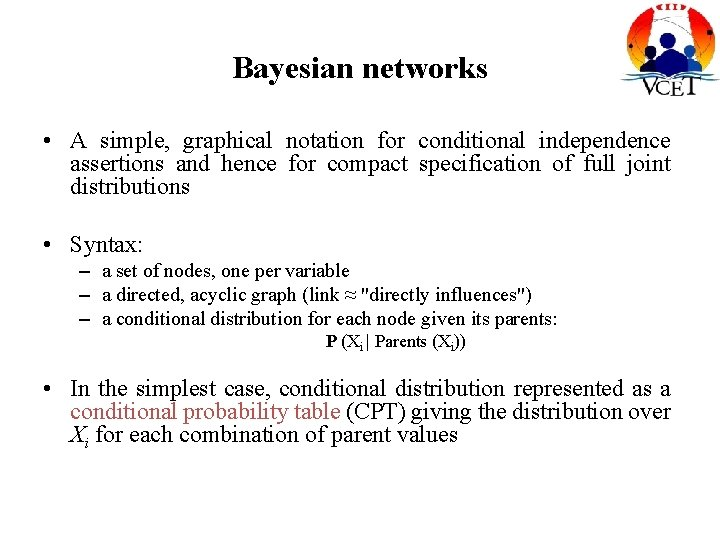Bayesian networks • A simple, graphical notation for conditional independence assertions and hence for compact specification of full joint distributions • Syntax: – a set of nodes, one per variable – a directed, acyclic graph (link ≈ "directly influences") – a conditional distribution for each node given its parents: P (Xi | Parents (Xi)) • In the simplest case, conditional distribution represented as a conditional probability table (CPT) giving the distribution over Xi for each combination of parent valuesExample • Topology of network encodes conditional independence assertions: • Weather is independent of the other variables • Toothache and Catch are conditionally independent given Cavity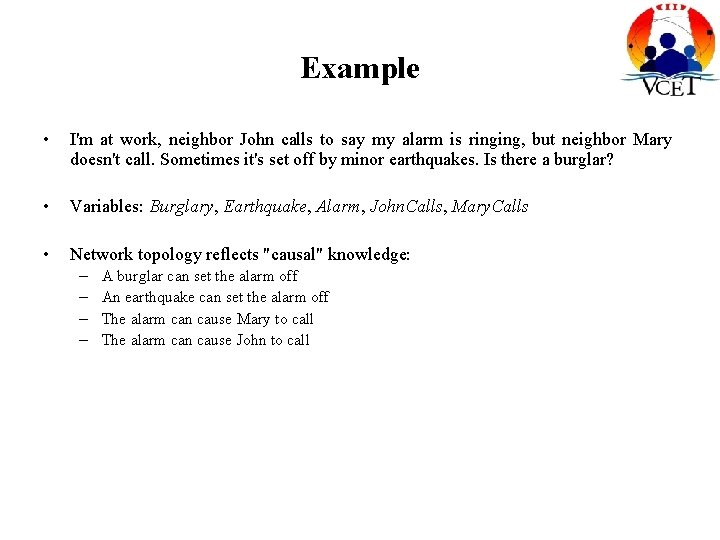Example • I'm at work, neighbor John calls to say my alarm is ringing, but neighbor Mary doesn't call. Sometimes it's set off by minor earthquakes. Is there a burglar? • Variables: Burglary, Earthquake, Alarm, John. Calls, Mary. Calls • Network topology reflects "causal" knowledge: – – A burglar can set the alarm off An earthquake can set the alarm off The alarm can cause Mary to call The alarm can cause John to call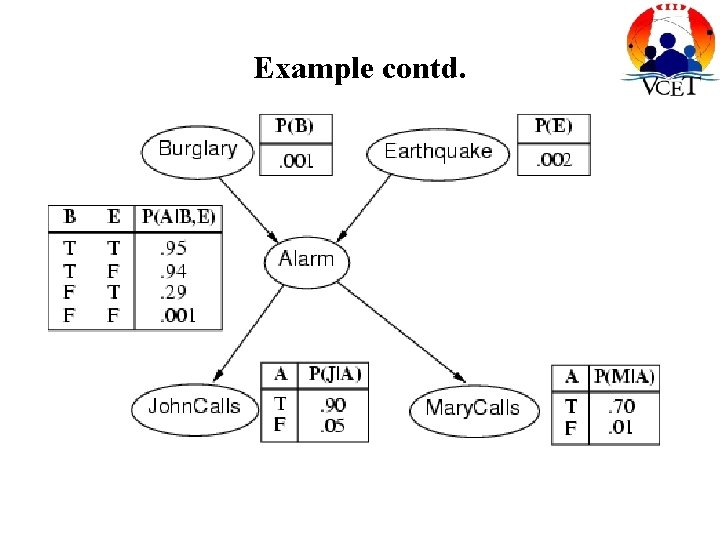Example contd.Compactness • A CPT for Boolean Xi with k Boolean parents has 2 k rows for the combinations of parent values • Each row requires one (the number for Xi = false is just 1 -p) • If each variable has no more than k parents, the complete network requires O(n · 2 k) numbers • I. e. , grows linearly with n, vs. O(2 n) for the full joint distribution • For burglary net, 1 + 4 + 2 = 10 numbers (vs. 25 -1 = 31) number p for Xi = true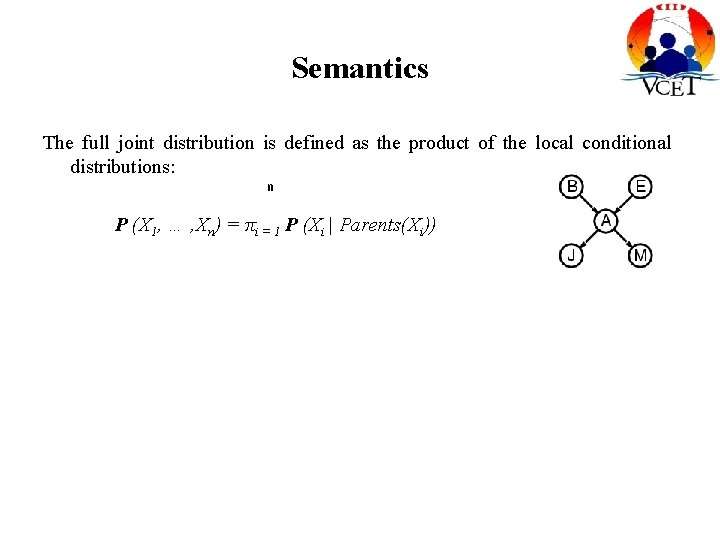Semantics The full joint distribution is defined as the product of the local conditional distributions: n P (X 1, … , Xn) = πi = 1 P (Xi | Parents(Xi))Constructing Bayesian networks • 1. Choose an ordering of variables X 1, … , Xn • 2. For i = 1 to n – add Xi to the network – select parents from X 1, … , Xi-1 such that P (Xi | Parents(Xi)) = P (Xi | X 1, . . . Xi-1) This choice of parents guarantees: n P (X 1, … , Xn) (chain rule) = πin=1 P (Xi | X 1, … , Xi-1) = πi =1 P (Xi | Parents(Xi)) (by construction)Example • Suppose we choose the ordering M, J, A, B, E P(J | M) = P(J)?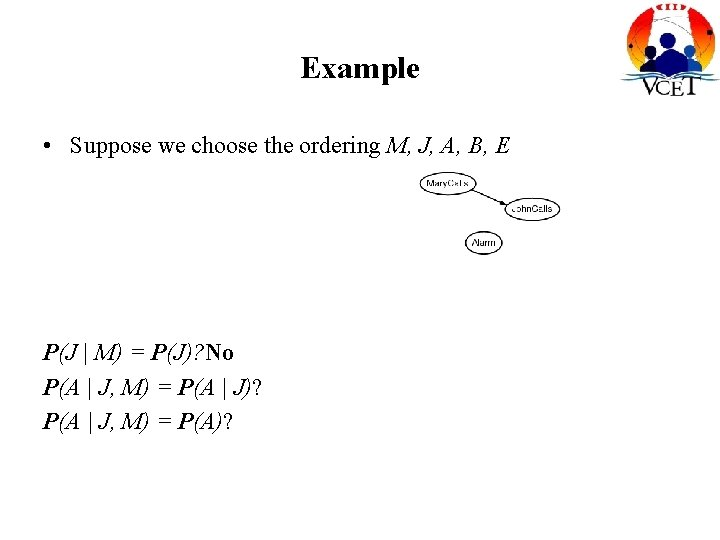Example • Suppose we choose the ordering M, J, A, B, E P(J | M) = P(J)? No P(A | J, M) = P(A | J)? P(A | J, M) = P(A)?Example • Suppose we choose the ordering M, J, A, B, E P(J | M) = P(J)? No P(A | J, M) = P(A | J)? P(A | J, M) = P(A)? No P(B | A, J, M) = P(B | A)? P(B | A, J, M) = P(B)?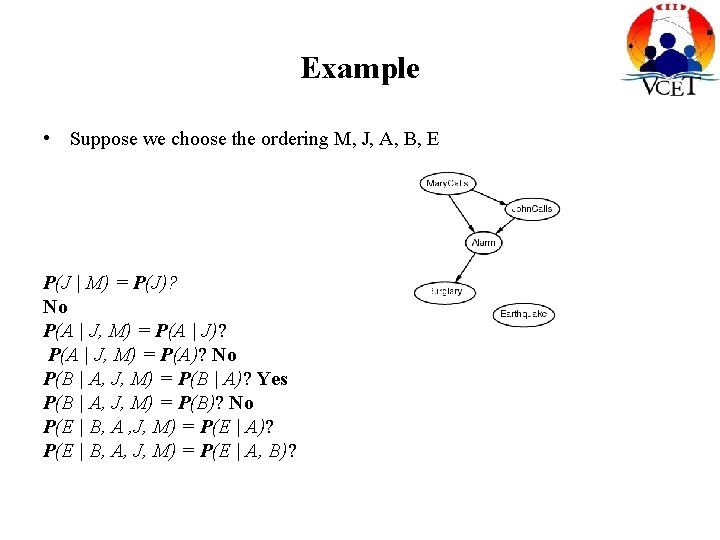Example • Suppose we choose the ordering M, J, A, B, E P(J | M) = P(J)? No P(A | J, M) = P(A | J)? P(A | J, M) = P(A)? No P(B | A, J, M) = P(B | A)? Yes P(B | A, J, M) = P(B)? No P(E | B, A , J, M) = P(E | A)? P(E | B, A, J, M) = P(E | A, B)?Example • Suppose we choose the ordering M, J, A, B, E P(J | M) = P(J)? No P(A | J, M) = P(A | J)? P(A | J, M) = P(A)? No P(B | A, J, M) = P(B | A)? Yes P(B | A, J, M) = P(B)? No P(E | B, A , J, M) = P(E | A)? No P(E | B, A, J, M) = P(E | A, B)? Yes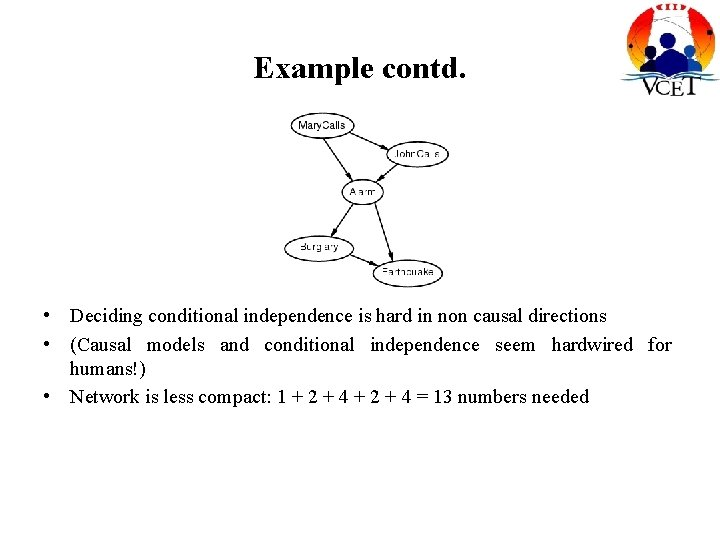Example contd. • Deciding conditional independence is hard in non causal directions • (Causal models and conditional independence seem hardwired for humans!) • Network is less compact: 1 + 2 + 4 = 13 numbers needed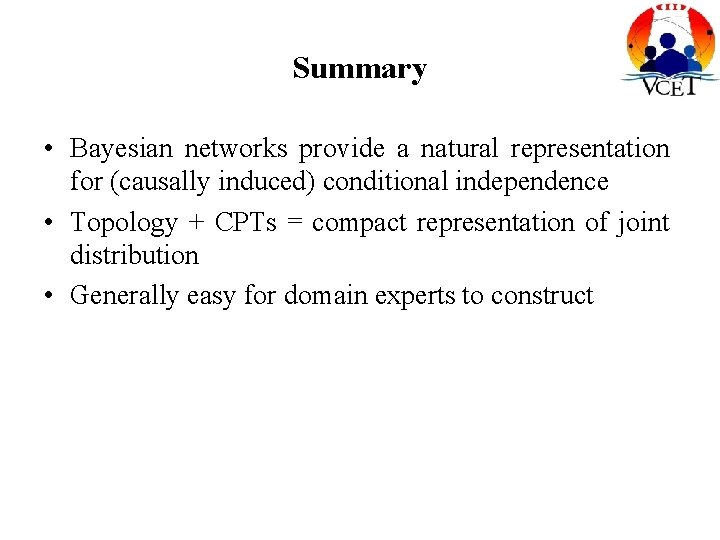Summary • Bayesian networks provide a natural representation for (causally induced) conditional independence • Topology + CPTs = compact representation of joint distribution • Generally easy for domain experts to construct